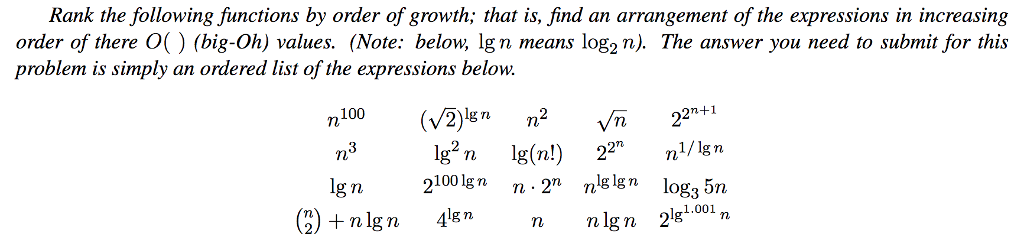# Question & Answer: ave the ordered list, I just want to understand how you will get the answer just the explanation…..I have the ordered list, I just want to understand how you will get the answer just the explanation that how you would order the big-o notation for all expression thanks

Rank the following functions by order of growth; that is, find an arrangement of the expressions in increasing Order of there。() (big-Oh) values. (Note: below, Ign means log2 n). The answer you need to submit for this problem is simply an ordered list of the expressions below. 2n+1 g n n lg n 4lgn 2)nlig n4*nnIgn 2«1.00 n nlgn 28001,n

Don't use plagiarized sources. Get Your Custom Essay on
Question & Answer: ave the ordered list, I just want to understand how you will get the answer just the explanation…..
GET AN ESSAY WRITTEN FOR YOU FROM AS LOW AS \$13/PAGE

Explanation :

Let me explain you the Definition of Big O : Given a function f(n) , there exist as function c*g(n) which is asymptotically higher then f(n) , such that c>0 and at some point n= n0, f(n)will be equal to g(n) but after

The idea is see

For example : f(n) = 2n^2 + 3 , then we can write g(n) = 3n^2 i.e f(n) = O(g(n))

Similarly
f(n) = 2^n + 2n^2 + 3 , then we can write g(n) = 2* 2^n i.e f(n) = O(g(n)) why we wrote because exponential functions has much much higher growth rate than polynomial, so its just the ordering We need to remember that

Exponential >>>> Polynomial

And Polynomial is much much greater than Logarithmic like n >logn, n^2 > nlogn etc

Similarly, Logarithmic is greater than constants Like log n > 100 etc

So, The order is

Exponential >>>> Polynomial >>>Logarithmic>>> Constants

Hence the Big Oh is the Upper bound of function hence we choose the one having higher growth rate

Example
f(n) = n^3 + n^2 + 3 , then we can write g(n) = 2n^3 i.e f(n) = O(g(n))

So the Big oh for the Function f(n) is O(n^3)